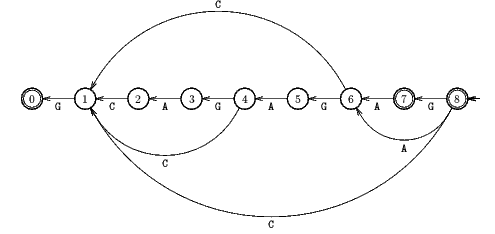Name
• String
• Substring
• Search
Edit

The Boyer-Moore type algorithms match some suffixes of the pattern but it is possible to match some prefixes of the pattern by scanning the character of the window from right to left and then improve the length of the shifts. This is make possible by the use of the suffix oracle of the reverse pattern. This data structure is a very compact automaton which recognizes at least all the suffixes of a word and slightly more other words The string-matching algorithm using the oracle of the reverse pattern is called the Backward Oracle Matching algorithm.

The suffix oracle of a word w is a Deterministic Finite Automaton O(w) =(Qq0TE).

The language accepted by O(w) is such that {u in* :  exists v in* such that w = vu} in L(O(w)).

The preprocessing phase of the Backward Oracle Matching algorithm consists in computing the suffix oracle for the reverse pattern xR. Despite the fact that it is able to recognize words that are not factor of the pattern, the suffix oracle can be used to do string-matching since the only word of length greater or equal m which is recognized by the oracle is the reverse pattern itself. The computation of the oracle is linear in time and space in the length of the pattern.

During the searching phase the Backward Oracle Matching algorithm parses the characters of the window from right to left with the automaton O(xR) starting with state q0. It goes until there is no more transition defined for the current character. At this moment the length of the longest prefix of the pattern which is a suffix of the scanned part of the text is less than the length of the path taken inO(xR) from the start state q0 and the last final state encountered. Knowing this length, it is trivial to compute the length of the shift to perform.

The Backward Oracle Matching algorithm has a quadratic worst case time complexity but it is optimal in average. On the average it performs O(n.(logm) / m) inspections of text characters reaching the best bound shown by Yao in 1979.

Main features:

version of the Reverse Factor algorithm using the suffix oracle of xR instead of the suffix automaton of xR;

fast in practice for very long patterns and small alphabets;

preprocessing phase in O(m) time and space complexity;

searching phase in O(mn) time complexity;

optimal in the average.

Example:

Preprocessing phaseOracle used by Backward Oracle Matching algorithm.

Searching phase

 G C A T C G C A G A G A G T A T A C A G T A C G * 8 7 2 G C A G A G A G

Shift by: 5 (8 - 3)

 G C A T C G C A G A G A G T A T A C A G T A C G * 8 7 6 5 4 3 2 1 - G C A G A G A G

Shift by: 7 (8 - 1)

 G C A T C G C A G A G A G T A T A C A G T A C G * 7 2 1 G C A G A G A G

Shift by: 7 (8 - 1)

The Backward Oracle Matching algorithm algorithm performs 17 character comparisons on the example.

C